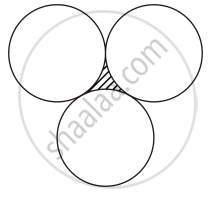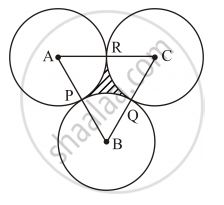Share

# In Figure 6, Three Circles Each of Radius 3.5 Cm Are Drawn in Such a Way that Each of Them Touches the Other Two. Find the Area Enclosed Between These Three Circles (Shaded Region). [ U S E π = 22 7 ] - Mathematics

Course

#### Question

In following figure, three circles each of radius 3.5 cm are drawn in such a way that each of them touches the other two. Find the area enclosed between these three circles (shaded region). ["Use" pi=22/7]#### Solution

The given information can be diagrammatically represented as follows:Here, A, B and C are the centres of the circles.

Radius of each circle, r = 3.5 cm

Thus, the measure of each of the sides of ΔABC is 3.5 cm + 3.5 cm = 7 cm.

Since the sides of triangle ABC are of equal lengths, it is an equilateral triangle.

∴ ∠A = ∠B = ∠C = 60°

Area of the shaded region = Area of ΔABC − (sum of areas of sectors APR, BPQ and CQR)

=sqrt3/4a^2-3xxO//360^@xxpir

=1.732/4xx(7"cm")^2-3xx60^@/360^@xx22/7xx3.5"cm"xx3.5"cm"

=21.217 "cm"^2-19.25cm^2

=1.97"cm"^2

Is there an error in this question or solution?